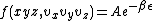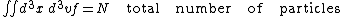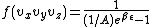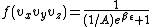# Kinetic theory of matter

Also found in: Dictionary, Thesaurus.

## Kinetic theory of matter

A theory which states that the particles of matter in all states of aggregation are in vigorous motion. In computations involving kinetic theory, the methods of statistical mechanics are applied to specific physical systems. The atomistic or molecular structure of the system involved is assumed, and the system is then described in terms of appropriate distribution functions. The main purpose of kinetic theory is to deduce, from the statistical description, results valid for the whole system. The distinction between kinetic theory and statistical mechanics is thus of necessity arbitrary and vague. Historically, kinetic theory is the oldest statistical discipline.Today a kinetic calculation refers to any calculation in which probability methods, models, or distribution functions are involved. See Statistical mechanics

Kinetic calculations are not restricted to gases, but occur in chemical problems, solid-state problems, and problems in radiation theory. Even though the general procedures in these different areas are similar, there are a sufficient number of important differences to make a general classification useful.

In classical ideal equilibrium problems there are no interactions between the constituents of the system. The system is in equilibrium, and the mechanical laws governing the system are classical. The basic information is contained in the Boltzmann distribution f (also called Maxwell or Maxwell-Boltzmann distribution) which gives the number of particles in a given momentum and positional range (d3x = dxdydz, d3v = dvxdvydvz, where x, y, and z are coordinates of position, and vx, vy, and vz are coordinates of velocity). In Eq. (1) ε is the energy, β =

(1)1/kT (where k is the Boltzmann constant and T is the absolute temperature), and A is a constant determined from Eq. (2). The
(2)calculations of gas pressure, specific heat, and the classical equipartition theorem are all based on these relations. See Boltzmann statistics

Many important physical properties refer not to equilibrium but to nonequilibrium states. Phenomena such as thermal conductivity, viscosity, and electrical conductivity all require a discussion starting from the Boltzmann transport equation. If one deals with states that are near equilibrium, the exact Boltzmann equation need not be solved; then it is sufficient to describe the nonstationary situation as a small perturbation superimposed on an equilibrium state.

The basic classical procedure for arbitrary systems (systems with interactions taken into account) that allows the calculation of macroscopic entities is that using the partition function.

Classical nonideal nonequilibrium theory is the most general situation that classical statistics can describe. In general, very little is known about such systems.

There are quantum counterparts to the classifications just described. In a quantum treatment a distribution function is also used for an ideal system in equilibrium to describe its general properties. For systems of particles which must be described by symmetrical wave functions, such as helium atoms and photons, one has the Bose distribution, Eq. (3), where β = 1/kT, and A is determined by Eq. (2). (3)See Bose-Einstein statistics

For systems of particles which must be described by antisymmetrical wave functions, such as electrons, protons, and neutrons, one has the Fermi distribution, Eq. (4).

(4)The application to electrons as an (ideal) Fermi-Dirac gas in a metal is the basis of the Sommerfeld theory of metals. See Fermi-dirac statistics, Free-electron theory of metals, Quantum statistics

McGraw-Hill Concise Encyclopedia of Physics. © 2002 by The McGraw-Hill Companies, Inc.
Site: Follow: Share:
Open / Close